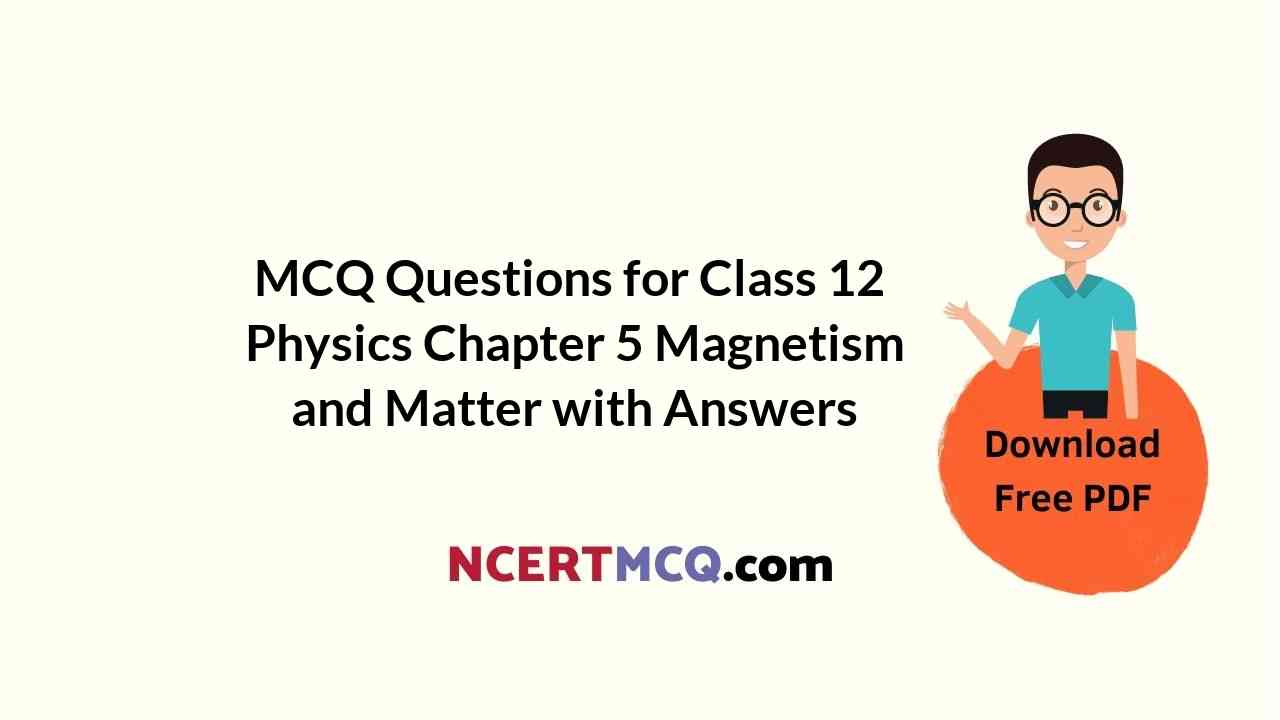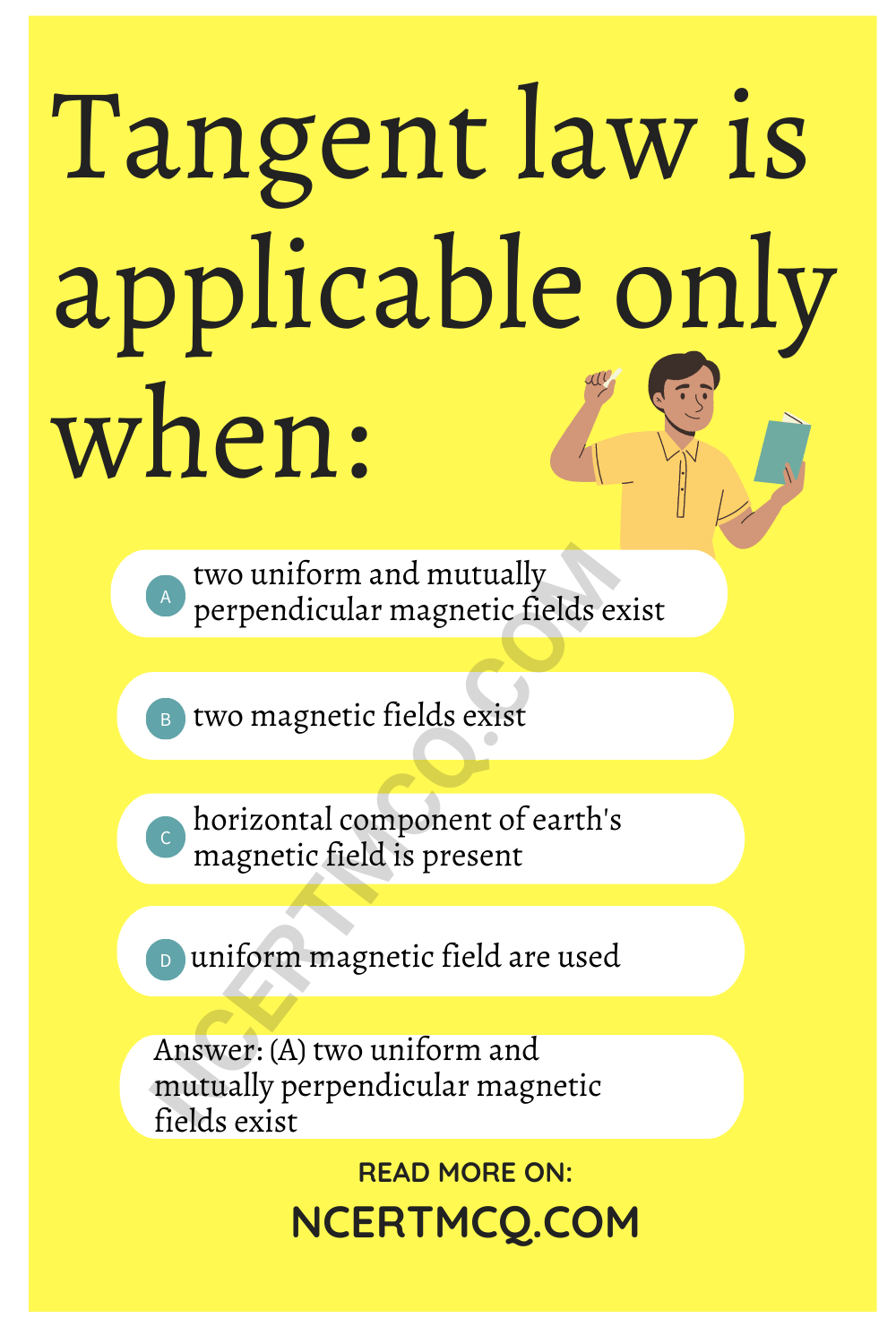Check the below NCERT MCQ Questions for Class 12 Physics Chapter 5 Magnetism and Matter with Answers Pdf free download. MCQ Questions for Class 12 Physics with Answers were prepared based on the latest exam pattern. We have provided Magnetism and Matter Class 12 Physics MCQs Questions with Answers to help students understand the concept very well.

## Magnetism and Matter Class 12 MCQs Questions with Answers

Magnetism And Matter Class 12 MCQ Question 1.
S.I. unit of flux is :
(a) Ohm
(b) Weber
(c) Tesla
(d) None

Class 12 Physics Chapter 5 MCQ Question 2.
What is the angle of dip at a place where the horizontal component of earth’s magnetic field is equal to the vertical component?
(a) 0°
(b) 30°
(c) 45°
(d) 90°

Magnetism And Matter MCQ Question 3.
Which of the following has a low value in ferrites?
(a) Conductivity
(b) Permeability
(c) Magnetic susceptibility
(d) All the above

Chapter 5 Physics Class 12 MCQ Question 4.
The dimensional representation of magnetic flux density is :
(a) [MLT-2]
(b) [MLT-2A-1]
(c) [MLT-2A-2]
(d) [MT-2A-1]

Ch 5 Physics Class 12 MCQ Question 5.
Tangent law is applicable only when:
(a) two uniform and mutually perpendicular magnetic fields exist
(b) two magnetic fields exist
(c) horizontal component of earth’s magnetic field is present
(d) uniform magnetic field are used

Answer: (a) two uniform and mutually perpendicular magnetic fields existMagnetism MCQ Class 12 Question 6.
Ferrites may be:
(a) ant. ferromagnetic
(b) ferromagnetic
(c) ferrimagnetic
(d) None of the above

Physics Class 12 Chapter 5 MCQ Question 7.
A magnetic bar of M magnetic moment is placed m the field of magnetic strength B, the torque acting on it is :
(a) $$\vec{M}$$.$$\vec{B}$$
(b) –$$\vec{M}$$.$$\vec{B}$$
(c) $$\vec{M}$$ × $$\vec{B}$$.
(d) $$\vec{B}$$ × $$\vec{M}$$

Answer: (c) $$\vec{M}$$ × $$\vec{B}$$.

MCQ On Magnetism Class 12 Question 8.
The magnetic lines of force inside a bar magnet:
(a) do not exist
(b) depends on area of cross-section of bar magnet
(c) are from N-pole to S-pole of the magnet
(d) are from S-pole to N-pole of the magnet.

Answer: (d) are from S-pole to N-pole of the magnet.

MCQ On Magnetism Class 12 Pdf Question 9.
A magnetic dipole moment is a vector quantity directed from:
(a) S to N
(b) N to S
(c) E to W
(d) W to E

MCQ Of Chapter 5 Physics Class 12 Question 10.
What is the magnetic field in the empty space enclosed by the toroidal solenoid of radius ‘R’? ,
(a) Infinity
(b) $$\frac{µ_0}{4π}$$.$$\frac{2πl}{R}$$
(c) $$\frac{µ_0}{4π}$$.($$\frac{πl}{R}$$)
(d) zero

Magnetism Class 12 MCQ Question 11.
A current carrying power line carries current from west to east. What will be direction of magnetic field 1 meter above it?
(a) N to S
(b) S to N
(c) E to W
(d) W to E

Class 12 Physics Ch 5 MCQ Question 12.
The dimensional representation of $$\sqrt{l/MB}$$ is similar to that of:
(a) frequency
(b) time
(c) distance
(d) speed

Magnetism And Matter Class 12 MCQ Questions Question 13.
On quadrupling the moment of inertia of a magnet, its frequency of oscillation will become:
(a) half
(b) double
(c) four times
(d) one-fourth

MCQ On Magnetism And Matter Question 14.
The magnetic field strength due to a short bar magnet directed along its axial line at a distance r is B. What is its value at the same distance along the equatorial line?
(a) B
(b) 2B
(c) $$\frac{B}{2}$$
(d) $$\frac{B}{4}$$

Answer: (c) $$\frac{B}{2}$$

Physics Chapter 5 Class 12 MCQ Question 15.
The neutral point in the magnetic field of a horizontally placed bar magnet is a point where the magnetic field due to that bar magnet is:
(a) zero
(b) more than that of earth
(c) less than that of earth
(d) equal to that of earth

Answer: (d) equal to that of earth

Question 16.
In a moving coil galvanometer, we use a radial magnetic field so that the galvanometer scale is :
(a) exponential
(b) linear
(c) algebraic
(d) logarithmic

Question 17.
The force between two parallel wire 2 × 10-7 Nm-1, placed 1 m apart to each other in vacuum. The electric current flowing through the wires is:
(a) 1 A
(b) zero
(c) 5 × 106 A
(d) 2 × 10-7 A

Question 18.
The force acting per unit length of a semi circular wire of radius R carrying a current I is:
(a) $$\frac{µ_0l^2}{4R}$$
(b) $$\frac{µ_0l^2}{2R}$$
(c) $$\frac{µ_0l^2}{R}$$
(d) $$\frac{2µ_0l^2}{R}$$

Answer: (a) $$\frac{µ_0l^2}{4R}$$

Question 19.
Which of the following has higher magnetic susceptibility?
(a) diamagnetic
(b) paramagnetic
(c) ferromagnetic
(d) None of these

Question 20.
The magnetic field of earth is due to:
(a) induction effect of the sun
(b) the presence of a large magnet at the centre of the earth
(c) interaction of cosmic rays with the current of earth
(d) motion and distribution of some material in an outside the earth

Answer: (d) motion and distribution of some material in an outside the earth

Question 21.
In a bar magnet, magnetic lines of force z :
(a) are produced only at north pole like rays of light from a bulb
(b) starts from north pole and ends at the south pole
(c) emerge in circular paths from the middle of the bar
(d) run continuously through the bar and outside

Answer: (b) starts from north pole and ends at the south pole

Question 22.
The Mariner’s compass is provided with Gimbals arrangement so as to :
(a) keep the needle always horizontal
(b) give a direct reading of declination
(c) give the direct value of dip
(d) all of the above

Answer: (c) give the direct value of dip

Question 23.
A wire of length I has a magnetic moment M. It is then bent into a semi-circular arc. The neyv magnetic moment is :
(a) M
(b) M.l.
(c) $$\frac{2M}{π}$$
(d) $$\frac{M}{π}$$

Answer: (c) $$\frac{2M}{π}$$

Question 24.
The relation between geometric length (L) and magnetic length (Lm) is:
(a) Lm = $$\frac{5}{6}$$ Lg
(b) Lm = $$\frac{6}{5}$$ Lg
(c) (a) Lm = Lg
(d) Lm = 2RY;UL Lg

Answer: (a) Lm = $$\frac{5}{6}$$ Lg

Question 25.
The radius of curvature of the path of charged particle in a uniform magnetic file is directly proportional to the
(a) charge on fie particle
(b) Momentum of particle
(c) energy of particle
(d) Strength of fieldQuestion 26.
The magnetic field at the centre of a current carrying circular loop is B. If the radius of the loop is doubled keeping the current unchanged, the magnetic field at the centre of loop will become:
(a) $$\frac{B}{2}$$
(b) $$\frac{B}{4}$$
(c) 2B
(d) 4B

Answer: (b) $$\frac{B}{4}$$